# Oops...

This website is made for modern browsers. You are seeing this, because your browser is missing a feature or two. Please install the latest update or switch to a modern browser. See you soon.

# Construction planes

#planes

Everything we draw in Rhino or Grasshopper is part of a global Cartesian coordinate system, also called the world coordinate system. Therefore, every geometric shape within this system possesses defined coordinates and we can use Euclidean transformations to change their position and orientation is space. But sometimes, it’s cumbersome to calculate the new coordinates. Also, when we draw a shape, its generation mechanism aligns it to the world coordinate system’s axes.

To ease both, the generation and transformation of geometric objects, there are construction planes, also called reference planes. Such a plane introduces a separate, local coordinate system and can be visioned as a drawing table set anywhere in space. For example, instead of creating a box in the world coordinate system and then rotate and move it to its destined position, we can also create a reference plane and then draw the box on this plane.Instead of creating a box and then positioning it (left), we can construct a reference plane and then create the box on it (right).

A lot of components in Grasshopper let us set a reference plane for either the creation of objects, transformations, or other operations. In general, it’s a better idea to generate construction planes first and then create the objects on top of them. In an algorithm, it’s easy to generate a unique plane for every object.Examples of components that use planes as input.

### Construct planes

The ribbon group Vector > Plane hosts various components to create planes. For example: XY Plane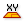XY Plane (XY)
Vector  >  Plane  >  XY Plane
World XY plane.
Inputs
Origin (O)Origin of plane
Outputs
Plane (P)World XY plane
creates a plane parallel to the world XY plane, but we can alter the origin of the coordinate system. With Construct Plane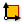Construct Plane (Pl)
Vector  >  Plane  >  Construct Plane
Construct a plane from an origin point and {x}, {y} axes.
Inputs
Origin (O)Origin of plane
X-Axis (X)X-Axis direction of plane
Y-Axis (Y)Y-Axis direction of plane
Outputs
Plane (Pl)Constructed plane
, we can set the origin and also the direction of the axes. Plane Normal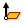Plane Normal (Pl)
Vector  >  Plane  >  Plane Normal
Create a plane perpendicular to a vector.
Inputs
Origin (O)Origin of plane
Z-Axis (Z)Z-Axis direction of plane
Outputs
Plane (P)Plane definition
creates a plane at the provided origin and perpendicular to the vector at Z.Selection of components to construct planes.

You can find a couple more components to create planes with various input parameters. The components are self-explanatory.

### Modify planes

The desired construction plane can also be obtained by altering an existing one. The components are hosted in the same ribbon group Vector > Plane. For example, we can modify the origin of an existing plane with Plane Origin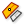Plane Origin (Pl Origin)
Vector  >  Plane  >  Plane Origin
Change the origin point of a plane
Inputs
Base (B)Base plane
Origin (O)New origin point of plane
Outputs
Plane (Pl)Plane definition
or change the orientation of the axes with Rotate Plane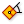Rotate Plane (PRot)
Vector  >  Plane  >  Rotate Plane
Perform plane rotation around plane z-axis
Inputs
Plane (P)Plane to rotate
Angle (A)Rotation (counter clockwise) around plane z-axis in radians
Outputs
Plane (P)Rotated plane
. Flip Plane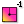Flip Plane (PFlip)
Vector  >  Plane  >  Flip Plane
Flip or swap the axes of a plane
Inputs
Plane (P)Plane to adjust
Reverse X (X)Reverse the x-axis direction
Reverse Y (Y)Reverse the y-axis direction
Swap axes (S)Swap the x and y axis directions
Outputs
Plane (P)Flipped plane
swaps the axes to our needs.Selection of components to alter planes.

Again, there are a couple more components that do all kinds of adjustments with provided planes.

### Deconstruct planes

The component Deconstruct Plane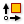Deconstruct Plane (DePlane)
Vector  >  Plane  >  Deconstruct Plane
Deconstruct a plane into its component parts.
Inputs
Plane (P)Plane to deconstruct
Outputs
Origin (O)Origin point
X-Axis (X)X-Axis vector
Y-Axis (Y)Y-Axis vector
Z-Axis (Z)Z-Axis vector
will dismantle a plane into its origin and axes.

### Construct planes with curves

Besides vectors, we can also derive planes from curves. With given curve parameters t, there are three option: Curve Frame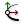Curve Frame (Frame)
Curve  >  Analysis  >  Curve Frame
Get the curvature frame of a curve at a specified parameter.
Inputs
Curve (C)Curve to evaluate
Parameter (t)Parameter on curve domain to evaluate
Outputs
Frame (F)Curve frame at {t}
creates a plane in which the tangent is the x-axis, Perp Frame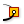Perp Frame (PFrame)
Curve  >  Analysis  >  Perp Frame
Solve the perpendicular (zero-twisting) frame at a specified curve parameter.
Inputs
Curve (C)Curve to evaluate
Parameter (t)Parameter on curve domain to evaluate
Outputs
Frame (F)Perpendicular curve frame at {t}
creates a plane in which the tangent is the z-axis and Horizontal Frame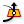Horizontal Frame (HFrame)
Curve  >  Analysis  >  Horizontal Frame
Get a horizontally aligned frame along a curve at a specified parameter.
Inputs
Curve (C)Curve to evaluate
Parameter (t)Parameter on curve domain to evaluate
Outputs
Frame (F)Horizontal curve frame at {t}
creates a plane that is parallel to the world xy plane and which has its origin at curve parameter t.

Instead of setting specific curve parameters, we can also use the related components Curve Frames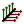Curve Frames (Frames)
Curve  >  Division  >  Curve Frames
Generate a number of equally spaced curve frames.
Inputs
Curve (C)Curve to divide
Count (N)Number of segments
Outputs
Frames (F)Curve frames
Parameters (t)Parameter values at division points
, Perp Frames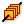Perp Frames (PFrames)
Curve  >  Division  >  Perp Frames
Generate a number of equally spaced, perpendicular frames along a curve.
Inputs
Curve (C)Curve to divide
Count (N)Number of segments
Align (A)Align the frames
Outputs
Frames (F)Curve frames
Parameters (t)Parameter values at frame points
and Horizontal FramesHorizontal Frames (HFrames)
Curve  >  Division  >  Horizontal Frames
Generate a number of equally spaced, horizontally aligned curve frames.
Inputs
Curve (C)Curve to divide
Count (N)Number of segments
Outputs
Frames (F)Curvature frames
Parameters (t)Parameter values at division points
. Here, we define at input N the number of segments in which the curve is divided into. The frames (planes) are created at each division point.

### Retrieve local coordinates

To find the local coordinates of a point on a construction plane, we can use Plane Coordinates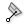Plane Coordinates (PlCoord)
Vector  >  Plane  >  Plane Coordinates
Get the coordinates of a point in a plane axis system.
Inputs
Point (P)Input point
System (S)Local coordinate system
Outputs
X coordinate (X)Point {x} coordinate
Y coordinate (Y)Point {y} coordinate
Z coordinate (Z)Point {z} coordinate
along with our point P and the construction plane at S.

### Retrieve planes from curves

For planar curves, we can use Planar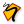Planar (Planar)
Curve  >  Analysis  >  Planar
Test a curve for planarity.
Inputs
Curve (C)Curve to evaluate
Outputs
Planar (p)Planarity of curve
Plane (P)Curve plane
Deviation (D)Deviation from curve plane
not only to verify the curve’s planarity, but also to find the plane that the curve is in. Unlike Curve Frame, which uses a tangent of the curve to construct the plane, Planar orients the plane to the world coordinate system.

### Retrieve planes from surfaces

To find the plane of a planar surface, we can use Is Planar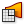Is Planar (Planar)
Surface  >  Analysis  >  Is Planar
Test whether a surface is planar
Inputs
Surface (S)Surface to test for planarity
Interior (I)Limit planarity test to the interior of trimmed surfaces
Outputs
Planar (F)Planarity flag of surface
Plane (P)Surface plane
to get the verification and the surface’s plane.

Using this component with a non-planar surface will return any plane, even when the surface was constructed with a base (construction) plane.

For non-planar surfaces, there is no unified plane and we need to evaluate a point on the surface to get the plane at this position. To do so, we use Evaluate Surface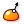Evaluate Surface (EvalSrf)
Surface  >  Analysis  >  Evaluate Surface
Evaluate local surface properties at a {uv} coordinate.
Inputs
Surface (S)Base surface
Point (uv){uv} coordinate to evaluate
Outputs
Point (P)Point at {uv}
Normal (N)Normal at {uv}
U direction (U)U direction at {uv}
V direction (V)V direction at {uv}
Frame (F)Frame at {uv}
and attach uv-coordinates that match the domain of the surface.

### Using Planes in Transformations

Besides the basic transformations mentioned in the beginning of this how-to, there are two powerful components to relocate geometric objects with reference planes. The first one is Move To Plane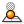Move To Plane (MoveToPlane)
Transform  >  Euclidean  >  Move To Plane
Translate (move) an object onto a plane.
Inputs
Geometry (G)Base geometry
Plane (P)Target plane
Above (A)Move when above plane
Below (B)Move when below plane
Outputs
Geometry (G)Translated geometry
Transform (X)Transformation data
: This component takes a geometry object G and a plane P as input and moves the objects onto the provided plane. At input A and B we can set a Boolean to false if objects on one side of the plane, above (A) or below (B), should not be moved. The objects are translated until the shape touches the plane.

The other component is Orient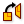Orient (Orient)
Transform  >  Euclidean  >  Orient
Orient an object. Orientation is sometimes called a 'ChangeBasis tranformation'. It allows for remapping of geometry from one axis-system to another.
Inputs
Geometry (G)Base geometry
Source (A)Initial plane
Target (B)Final plane
Outputs
Geometry (G)Reoriented geometry
Transform (X)Transformation data
and it resembles a move and rotate transformation. We need to provide the geometric objects G and their initial (source) planes at A. At input B we set the target plane(s). The coordinates of the object are then remapped to the new plane. This is helpful to reposition and arrange objects independent of their previous relation.

This page is open source. Edit it on GitHub or see how you can contribute.